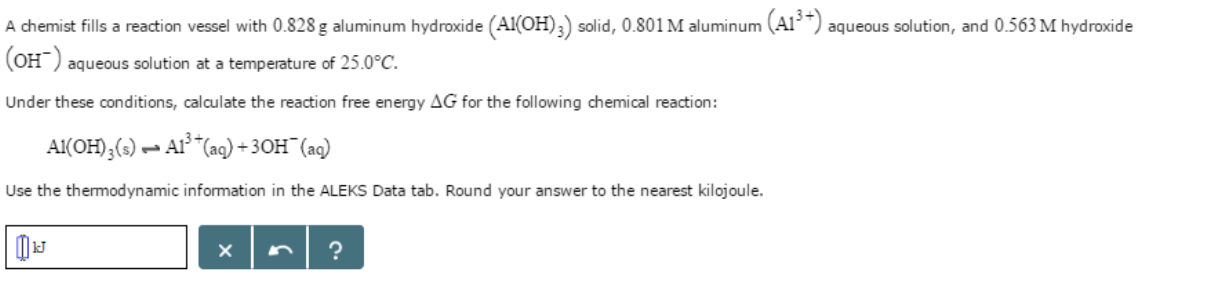# Problem: A chemist fills reaction vessel with 0.828g aluminum hydroxide (Al OH)3) solid, 0.801 M aluminum (Al3+) aqueous solution, and 0.563M hydroxide (OH-) aqueous solution at a temperature of 25.0° C. Under these conditions, calculate the reaction free energy ΔG for the following chemical reaction:Al(OH)3(s) ⇌ AI3+(aq) + 3OH-(aq)Round your answer to the nearest kilojoule.

###### FREE Expert Solution
100% (378 ratings)###### Problem Details

A chemist fills reaction vessel with 0.828g aluminum hydroxide (Al OH)3) solid, 0.801 M aluminum (Al3+) aqueous solution, and 0.563M hydroxide (OH-) aqueous solution at a temperature of 25.0° C. Under these conditions, calculate the reaction free energy ΔG for the following chemical reaction:

Al(OH)3(s) ⇌ AI3+(aq) + 3OH-(aq)